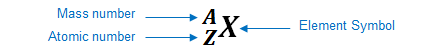# Problem: How do scientists determine the number of neutrons in an isotope of an atom?A. They find the number of protons.B. They divide the atomic mass by two.C. They add the number of electrons and protons.D. They subtract the atomic number from the atomic mass.

###### FREE Expert Solution

We’re being asked to determine the name of the element that has an atomic number of 59.

An element can be represented as:You can determine the identity of the element using its atomic number:

Atomic number (Z):

85% (156 ratings)###### Problem Details

How do scientists determine the number of neutrons in an isotope of an atom?

A. They find the number of protons.

B. They divide the atomic mass by two.

C. They add the number of electrons and protons.

D. They subtract the atomic number from the atomic mass.

Frequently Asked Questions

What scientific concept do you need to know in order to solve this problem?

Our tutors have indicated that to solve this problem you will need to apply the Subatomic Particles concept. You can view video lessons to learn Subatomic Particles. Or if you need more Subatomic Particles practice, you can also practice Subatomic Particles practice problems.